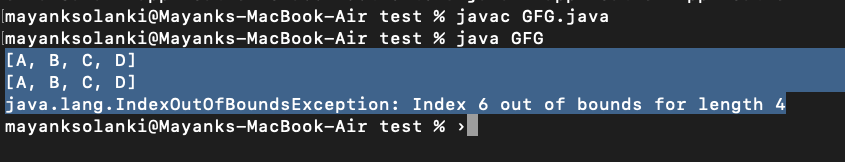How to Replace a Element in Java ArrayList?

• Difficulty Level : Basic
• Last Updated : 27 Jul, 2021

To replace an element in Java ArrayList, set() method of java.util. An ArrayList class can be used. The set() method takes two parameters-the indexes of the element which has to be replaced and the new element. The index of an ArrayList is zero-based. So, to replace the first element, 0 should be the index passed as a parameter.

Declaration:

public Object set(int index, Object element)

Return Value: The element which is at the specified index

Exception Throws: IndexOutOfBoundsException
This occurs when the index is out of range.

index < 0 or index >= size()

Implementation:

Here we will be proposing out 2 examples wherein one of them we will be setting the index within bound and in the other, we will be setting the index out of bounds.

Example 1: Where Index is Within Bound

Java

 // Java program to demonstrate set() Method of ArrayList// Where Index is Within Bound // Importing required classesimport java.io.*;import java.util.*; // Main classclass GFG {   // Main driver method  public static void main(String[] args) {     // Try block to check for exceptions    try {       // Creating an object of Arraylist class      ArrayList list = new ArrayList<>();        // Adding elements to the List      // using add() method       // Custom input elements      list.add("A");      list.add("B");      list.add("C");      list.add("D");       // Print all the elements added in the above object      System.out.println(list);       // 2 is the index of the element "C".      //"C" will be replaced by "E"      list.set(2, "E");       // Printing the newly updated List      System.out.println(list);     }     // Catch block to handle the exceptions    catch (Exception e) {       // Display the exception on the console      System.out.println(e);    }  }}
Output
[A, B, C, D]
[A, B, E, D]

Example 2: Where Index is Out of Bound

Java

 // Java program to demonstrate set() Method of ArrayList// Where Index is Out of Bound // Importing required classesimport java.io.*;import java.util.*; // Main classclass GFG {   // Main driver method  public static void main(String[] args) {     // Try block to check for exceptions    try {       // Creating an object of Arraylist class      ArrayList list = new ArrayList<>();        // Adding elements to the List      // using add() method       // Custom input elements      list.add("A");      list.add("B");å      list.add("C");      list.add("D");       // Print all the elements added in the above object      System.out.println(list);              // Settijg the element at the 6 th index which      // does not exist in our input list object      list.set(6);         // Printing the newly updated List      System.out.println(list);    }     // Catch block to handle the exceptions    catch (Exception e) {       // Display the exception on the console      System.out.println(e);    }  }}

Output:My Personal Notes arrow_drop_up